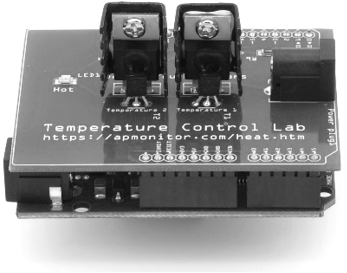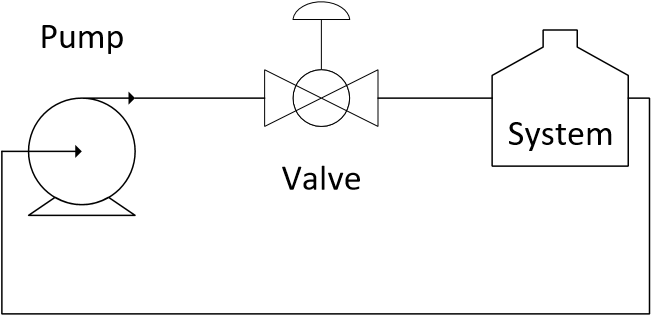## Quiz on Flow Valves1. A fail-open valve is the same as a(n):

A. Air-to-open valve
Incorrect. Air pressure opens the valve. The absence of air pressure closes the valve.
B. Air-to-close valve
Correct. Air pressure closes the valve. The absence of this air pressure opens the valve.
C. Fail-close valve
Incorrect. When the valve fails, pressure is released and closes the valve.
D. Globe valve
Incorrect. A globe valve may be designed to be either fail-open or fail-close

2. Why is it desirable to maintain a linear relationship between valve lift and flow rate? Select three correct answers without selecting the one that is incorrect.

A. Valve does not wear out as fast
Incorrect. Linearity does not affect valve stem travel. If there is excessive valve movement then it can wear out the components such as the seals or motor
B. It avoids hyper-sensitive regions where small changes in valve output lead to large changes in flow.
Correct. Nonlinear relationship between lift and flow means that the valve changes will either cause large or small changes in flow depending on whether the valve is mostly opened or closed.
C. It avoids insensitive regions where large changes in valve output lead to small changes in flow.
Correct. Nonlinear relationship between lift and flow means that the valve changes will either cause large or small changes in flow depending on whether the valve is mostly opened or closed.
D. Harder to tune a PID flow controller that functions well over the range of valve operation
Correct. A linear relationship means that one PID controller gain K_c will be tuned correctly over the entire range of operation for the flow controller. Recall that IMC tuning with no dead-time recommends K_c = 1/K_p. If the lift to flow is linear then the process gain K_p is the same throughout the range of operation such as 5% open or 95% open.

3. What is one way to create a linear relationship between valve lift and flow rate?

A. Implement PID gain (K_c) scheduling
Incorrect. PID gain scheduling is a method to compensate for a nonlinear relationship by changing the controller gain K_c based on the PV measurement. This extra complication for a flow controller can be avoided by selecting a valve trim that creates a linear relationship between lift and flow.
B. Adjust C_v value
Incorrect. This does affect the relationship between lift and flow but only as a scalar. It changes the slope of lift versus flow but does not correct for nonlinearity.
C. Modify the trim
Correct. The valve trim can be changed to another shape to create a linear relationship between lift and flow. The process pressure drop is often a quadratic function of flow. The equal percentage valve trim is commonly used to compensate for this nonlinear (quadratic) relationship.
D. Adjust the pressure drop across the valve (\Delta P_v)
Incorrect. \Delta P_v is not a design variable. It depends on the valve lift, flow, and valve design.

4. Pump pressure is dissipated across the system and valve that maintains the flow rate through the system.$$\Delta P_{pump} = \Delta P_{valve} + \Delta P_{system}$$

The pump \Delta P_{pump} generates 50 bar of pressure. The pressure drop across the system (not including the valve) has a quadratic relationship with flow \Delta P_{system} = 2 q^2 bar where q has units of m^3/{hr}. If the flow through the valve and system is q = 4.4 m^3/{hr}, what is the pressure drop across the valve \Delta P_{valve}?

A. 38.72 bar
Incorrect. This is the system pressure drop, not the pressure drop across the valve.
B. 14.58 bar
Incorrect. This is the correct range for the valve pressure drop, between 1/4 and 1/3 of the total pressure drop. If the fractional pressure drop is too high then the C_v should be increased with a larger valve.
C. 11.28 bar
Correct. \DeltaP_{valve} = \DeltaP_{pump} - \DeltaP_{system} = 50 - 2 (4.4)^2 = 11.28 bar
D. 17.24 bar
Incorrect. This is the correct range for the valve pressure drop, between 1/4 and 1/3 of the total pressure drop. If the fractional pressure drop is too high then the C_v should be increased with a larger valve.